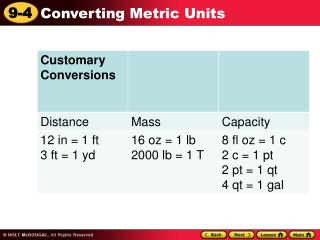DownloadDownload Presentation1 cm = 10 mm

# 1 cm = 10 mm

Télécharger la présentation## 1 cm = 10 mm

- - - - - - - - - - - - - - - - - - - - - - - - - - - E N D - - - - - - - - - - - - - - - - - - - - - - - - - - -
##### Presentation Transcript

1. Metric Measurements Distance Mass Capacity 1 km = 1,000 m 1 kg = 1,000 g 1 L = 1,000 mL 1 m = 100 cm 1 g = 1,000 mg 1 cm = 10 mm Convert metric measures by using a conversion factor or using proportions.

2. How large is a millimeter? The width of a pin from “Math Flash Measurement I” by M. and B. Yuskaitis

3. How large is a centimeter? The width of the top of your finger from “Math Flash Measurement I” by M. and B. Yuskaitis

4. How large is a meter? About the width of one & 1/2 doors 1 meter from “Math Flash Measurement I” by M. and B. Yuskaitis

5. How large is a kilometer? Whitmore A little over 1/2 of a mile 1 kilometer Walter White from “Math Flash Measurement I” by M. and B. Yuskaitis

6. How large is a milliliter? About a drop of liquid from “Math Flash Measurement I” by M. and B. Yuskaitis

7. How large is a liter? Half of a large pop bottle 1 liter from “Math Flash Measurement I” by M. and B. Yuskaitis

8. How heavy is a gram? A paper clip weighs about 1 gram from “Math Flash Measurement I” by M. and B. Yuskaitis

9. How heavy is a kilogram? A kitten weighs about 1 kilogram from “Math Flash Measurement I” by M. and B. Yuskaitis

10. Time Conversions 1 year (yr) = 365 days 1 year = 12 months (mo) 1 year = 52 weeks 1 week = 7 days 1 day = 24 hours (hr) 1 hour = 60 minutes (min) 1 minute = 60 seconds (s)

11. Helpful Hint To multiply by a power of 10, move the decimal to the right; to divide by a power of 10, move the decimal to the left.

12. Ex 1 Convert 450 g to _____ kg. 450 gx kg 1,000 g m 1 kg Write a proportion. = 1,000x = 450 kg The cross products are equal. Divide both sides by 1,000 to undo the multiplication. x = 0.45 kg

13. Ex 2 Convert. 8,500 g = _____ kg 8,500 gtx kg 1,000 g m 1 kg Write a proportion. = The cross products are equal. Divide both sides by 1,000 to undo the multiplication. 1,000x = 8,500 kg x = 8.5 kg

14. Measurement Conversions (Metric and Customary): 1. 12m = _________km 2. 12m = _________mm 3. 12m = _________cm 4. 48 in. = _________ft. 5. 48 in. = _________ yd. 6. 48 in. = _________ mile